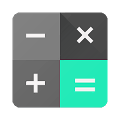Calculator provides simple and advanced mathematical functions in a beautifully designed app.• Perform basic calculations such as addition, subtraction, multiplication, and division
• Do scientific operations such as trigonometric, logarithmic, and exponential functions
• Perform basic calculations on the go using Android Wear

#### What’s New

• Edit existing formulas with cursor support on devices running Android version 6.0+
• Android O support

Name
Calculator
Package